The CKN Knowledge in Practice Centre is in the early stages of content creation and currently focuses on the theme of thermal management.
We appreciate any feedback or content suggestions/requests using the links below

# Heat transfer - A132

Heat transfer
Foundational knowledge article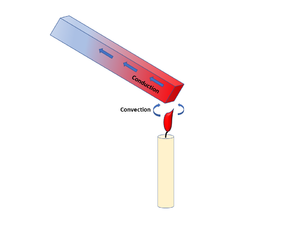Document Type Article
Document Identifier 132
Themes
Relevant Class

Material

Tags
Prerequisites

## Introduction

Heat transfer is the physics process responsible for the redistribution of thermal heat energy between systems. For composites, this includes the redistribution of heat both within the laminate itself, and between the laminate body and its surrounding environment. The heat transfer process has important roles in both the composite manufacturing process and the operational service of the composite material.

## Scope

This page introduces the fundamental topic of heat transfer and its application to composites processing. It describes the three main mechanisms of heat transfer, i.e. thermal conduction, thermal convection and thermal radiation, outlines the energy equation, measurement techniques, and the modelling of heat transfer in process simulation.

## Significance

Heat transfer is a fundamental component of the composite manufacturing process. In many cases, sufficient amounts of heat must be imposed on the laminate during the manufacturing process for its physical, and in certain cases chemical transformation into the final composite part.

Precise control of the temperature imposed on the laminate during manufacturing plays an important role in the following aspects:

• Thermoset matrix: ensuring a suitable level of cure is achieved for the intended mechanical properties. At the same time, tight control of temperature is critical during processing to control the evolution of cure and mitigate the development of defects due too little or too much resin flow
• Thermoplastic matrix: ensuring sufficient heating for melting and flow, and cooling for recrystallization
• All matrix types: influencing the microstructure distribution of the reinforcement fibres and matrix tougheners, morphology of matrix tougheners, final matrix porosity

## Prerequisites

Recommended documents to review before, or in parallel with this document:

## Overview

### Heat Transfer Mechanisms

Fundamentally, heat transfer is organized into three main mechanisms:

• Conduction
• Convection
• Radiation (considered negligible in a majority of composites processing scenarios)

For a system, heat transport can involve multiple mechanisms. Using the classic example of a metal rod being heated over a flame; the heat being transferred from the flame to the rod is considered to be by convection, while the heat transferred along the rod itself is by conduction . Applying this analogy to the composites processing scenario for a laminate curing in an oven or autoclave environment; the hot air transfers heat by convection, while the heat transfer between the tool and laminate part, and within the laminate itself is then by conduction.

#### Conduction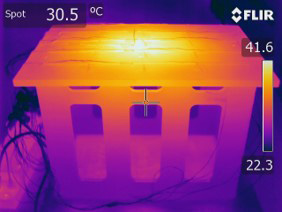Example of conductive heat transfer. The temperature within the tool is redistributed by conduction mechanisms.

Conduction is a heat transfer mode associated with the microscopic collisions between high energy particles and adjacent lower energy particles causing a redistribution of the kinetic energy within a material or medium. The heat flow is proportional to the temperature gradient imposed and the intrinsic properties of the material. Conduction is considered the primary heat transfer mechanism in solid materials.

#### Convection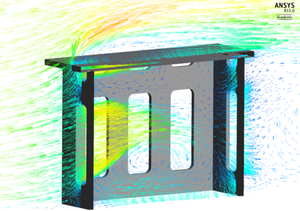Example of convective heat transfer. Heat is transferred to the part by hot air moving over the surface.

Convection is a heat transfer mode driven by the bulk motion of a fluid. Energy transport by convection (e.g. heat) results from the combination of two heat transfer mechanisms: conduction and the fluid flow process of advection. Convection takes place in the presence of fluid motion and can occur by forced fluid motion (forced convection) or by natural fluid motion (natural convection). In composites processing, convection is an important heat transfer mechanism occurring in oven and autoclave processing methods.

There are two types of convection transport: forced convection and free convection. These two modes of convection differ by the driving force leading the fluid flow:

##### Forced Convection

In forced convection, the motion of the fluid is governed by external mechanical forces and typically controlled in production by a pump or a fan.  For heating equipment like autoclaves or ovens, flowing gas driven by a fan is used to heat the part-tool assembly.

##### Natural Convection

In free convection, also known as natural convection, the motion of the fluid is driven by the temperature gradients in the fluid without external input. This occurs for open-air processes without a fan, where air movement may develop due to temperature-induced buoyant forces acting on the air. Similarly, a hot part naturally cooling in an open air environment is an example of natural convection heat transfer taking place.

In composites processing, conduction and convection are the dominant heat transfer mechanisms and thermal radiation is generally negligible .

## Heat Transfer Interactions During Composites Processing

Heat plays a critical role in the composites manufacturing process, controlling resin flow, cure and crystallization kinetics, and even the physical distribution and morphology of the various laminate constituents. During processing, heat is transferred between the laminate, tooling and manufacturing equipment. Heat transfer in composites processing is a systems problem, where the laminate part, tool, and heating equipment all interact together. During heated manufacturing processes, thermal heat is transferred from the manufacturing equipment to the laminate to form the final part. For thermoset matrix composites (e.g. polyester, epoxy) the heat is required for the curing of the thermoset resin, while for thermoplastic matrix composites the heat is required for melting (flow) and recrystallization (solidification) of the matrix.

### Example of Thermal Process Interactions

Two typical processing examples illustrating the role of heat transfer in the composite manufacturing process are given below (for further details of the interactions outlined, please see the Systems Interactions page):

Manufacturing Process Heat Transfers Involved

#### Hot Press

• Press platen –> part [conduction]
• Internal laminate heat redistribution [conduction]

#### Autoclave and Convection Oven

• Gaseous environment –> part (via consumables) [convection]
• Gaseous environment –> tool [convection]
• Tool –> part [conduction]
• Internal laminate heat redistribution [conduction]

### System Heat Sources and Heat Sinks

Like water flowing downstream, thermal heat flows from regions of high thermal energy (warmer temperature) to regions of lower thermal energy (cooler temperature). Whether a laminate heats up or cools down depends on the temperature gradient present between the laminate and the surrounding system. The thermal heat energy flowing into or out of the laminate can be described by considering the potential heat sources (heat input) and heat sinks (heat removal) in the system.

• Equipment with a heat source
• Tool when hot
• Material when generating heat (i.e. exothermic curing reaction of a thermoset resin)

#### Heat Sinks (removes heat)

• Equipment with a cooling system
• Tool when cold
• Material when absorbing heat (i.e. melting of a thermoplastic resin)

All system objects can act as either a heat source or a heat sink. Whether an object is a source or a sink is dependent upon the object's temperature in comparison to other surrounding objects in its environment.

## Modelling

Heat transfer can be modelled to various levels of complexity, from 0-D (no part thickness or dimensional considerations) up through 3-D.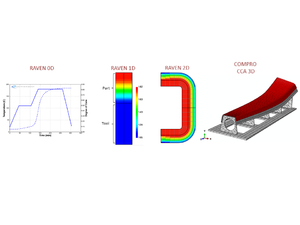Examples of thermal modelling possible with varying levels of complexity (increasing spatial dimensions). Images produced from simulations using commercial software RAVEN and COMPRO by Convergent Manufacturing Technologies.

The necessary number of dimensions to obtain sufficient accuracy is dependent on the geometry of the part, and the analysis being performed. For some cases, 1-D modelling is sufficient, while in other cases 2-D and 3-D modelling may be required.

In many cases, the typical laminate part aspect ratio (thickness is order of magnitudes less in comparison to length and depth dimensions) and part geometry generally allows for simple 1-D modelling of the through-thickness thermal distribution to often be sufficient.

### The Energy Equation

The heating of a laminate can be simplified and described as a heat energy balance, where$Rate\ of\ heat\ increase = Rate\ of\ heat\ addition\ by\ conduction$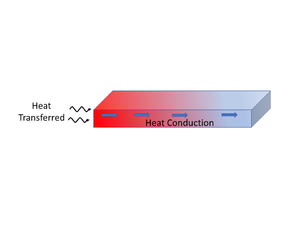Illustration of heat balance distribution. Heat transferred to laminate (heat energy increase) = laminate heat conduction contributions.

Modelled, this can be presented as the following general heat balance equation$\frac{\partial}{\partial t}(\rho c_pT)= \frac{\partial}{\partial x}\Bigl(k_{xx}\frac{\partial T}{\partial x}\Bigr)+\frac{\partial}{\partial y}\Bigl(k_{yy}\frac{\partial T}{\partial y}\Bigr)+\frac{\partial}{\partial z}\Bigl(k_{zz}\frac{\partial T}{\partial z}\Bigr)$

Breaking down the general heat balance equation by the individual heat components,

$$\frac{\partial}{\partial t}(\rho c_pT)=$$ Heat accumulation in the laminate

$$\frac{\partial}{\partial x}\Bigl(k_{xx}\frac{\partial T}{\partial x}\Bigr)+ ... =$$ Conduction heat transfer contributions

Where,

$$t =$$ Time [s]

$$\rho =$$ Density [kg/m3]

$$c_p =$$ Specific heat capacity [J/kg·K]

$$T =$$ Temperature [K]

$$k =$$ Thermal conductivity (in principle direction x, y, or z) [W/m·K]

$$x,\, y,\, z =$$ Respective principle direction x, y, or z

The rate of heat transferred into the system is provided by the heating equipment or environment (room temperature cure). If this heat transfer boundary condition is convective in nature (moving air), the heating rate can be described by$\dot Q = hA\Delta T$

Where,

$$h =$$ Convective heat transfer coefficient [W/m2·K]

$$A =$$ Surface area [m2]

$$\Delta T =$$ Change in temperature [K]

During the curing cycle for thermosets, internal heat is generated by the exothermic cross-linking reaction. In this scenario, the heat balance equation gains an additional $$\dot Q_r$$ term (reaction heating rate)$\frac{\partial}{\partial t}(\rho c_pT)= \frac{\partial}{\partial x}\Bigl(k_{xx}\frac{\partial T}{\partial x}\Bigr)+\frac{\partial}{\partial y}\Bigl(k_{yy}\frac{\partial T}{\partial y}\Bigr)+\frac{\partial}{\partial z}\Bigl(k_{zz}\frac{\partial T}{\partial z}\Bigr)+\dot Q_r$

The internal heat generation modelled as$\dot Q_r = \frac{d\ x}{dt}(1-V_f)\rho_r H_R$

Where,
$$\frac{d\ x}{dt} =$$ Rate change of degree of cure [1/s]

$$V_f =$$ Volume fraction fibre

$$\rho_r =$$ Density of resin [kg/m3]

$$H_R =$$ Total heat of reaction [J/kg]

### 1-D Thermal Modelling Example

For many cases, 1-D modelling of the through-thickness thermal distribution is sufficient. Provided here is an example for the curing of a thermoset matrix laminate modelled 1-D in the through-thickness direction. Shown are the heat transfer interactions, temperature profile, and heat equation involved for the lay-up stack for the heating stage, and after the thermoset exothermic reaction has kicked off with internal generated heat being released. If a substantial amount of heat is generated, the heat may leave the laminate and transfer into its surrounding environment.

#### Example: Autoclave cured carbon fibre/epoxy prepreg laminate

• Scenario Setup:
Laminate 1-D (through-thickness) Lay-up Stack Temperature History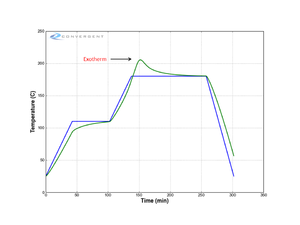Example of a typical temperature history of a laminate following a manufacturer's recommended cure cycle (MRCC). Note the hump in laminate temperature due to the thermoset resin exothermic reaction.

• 1-D Thermal Modelling Representation:
Temperature Profile Heat Transfer System Interactions Heat Equations

#### Laminate Heat Up

Heat Transfer Balance:

Air/Part Interface

Rate of heat transfer by convection$\dot Q = hA\Delta T$

Laminate and Tool Heat Balance

Laminate and tool energy balance$\frac{\partial}{\partial t}(\rho c_pT)= \frac{\partial}{\partial z}\Bigl(k_{zz}\frac{\partial T}{\partial z}\Bigr)$

Air/Tool Interface

Rate of heat transfer by convection$\dot Q = hA\Delta T$

#### Laminate Exotherm

Heat Transfer Balance:

Air/Part Interface

Rate of heat transfer by convection$\dot Q = hA\Delta T$

Laminate and Tool Heat Balance

Laminate energy balance$\frac{\partial}{\partial t}(\rho c_pT)= \frac{\partial}{\partial z}\Bigl(k_{zz}\frac{\partial T}{\partial z}\Bigr)+\dot Q_r$

Internal heat generation (laminate exotherm)$\dot Q_r = \frac{d\ x}{dt}(1-V_f)\rho_r H_R$

Tool energy balance$\frac{\partial}{\partial t}(\rho c_pT)= \frac{\partial}{\partial z}\Bigl(k_{zz}\frac{\partial T}{\partial z}\Bigr)$

Air/Tool Interface

Rate of heat transfer by convection$\dot Q = hA\Delta T$

## Measurement

The heat transfer process can be measured by proxy in two ways:

1. Temperature measurement: tracking temperature evolution (with time and position) to develop an understanding of the thermal management system and when available to validate a thermal model
2. Heat transfer coefficients: measuring the heat transfer coefficients, conduction $$k$$ and convection $$h$$ to use them for thermal modelling as inputs

Listed below are the different techniques to measure temperature and heat transfer coefficients.

### Temperature Measurement

• Thermocouple
• Infrared Camera
• Fibre Optic

### Heat Transfer Coefficients

#### Convective Heat Transfer Coefficient

• The following table from Slesinger et al. , provides a list of the possible methods available to measure airflow and convective heat transfer coefficients (HTC).

##### Airflow and heat transfer measurement methods. Source: 
Anemometers • Measures actual velocity values

• Non-directional

• Noisy results (turbulence) • Expensive, difficult to position • Local – need to know where

Calorimeters • Inexpensive

• Provides representative HTC values

• Time consuming data reduction

• Non-directional • Length scale dependency (real HTC may be different) • Local - need to know where

CFD • Shows velocity magnitude and direction

• All areas can be evaluated • Parametric evaluation

• Model may lack realistic detail

• Time consuming, expensive • Requires skilled personnel • Must be validated experimentally

Instrumented Part • Provides actual HTC • Accuracy limited by number of thermocouples

• Time consuming data reduction •Autoclave load or part geometry changes require new test • Non-directional • Local - need to know where

Video • Inexpensive

• Intuitive to analyze • Large areas can easily be monitored

• Qualitative

• Limited to ambient temperature and pressure

## Related pages

Introduction to Composites Articles
Foundational Knowledge Articles
Foundational Knowledge Method Documents
Foundational Knowledge Worked Examples
Systems Knowledge Articles
Systems Knowledge Method Documents
Systems Knowledge Worked Examples
Systems Catalogue Articles
Systems Catalogue Objects – Material
Systems Catalogue Objects – Shape
Systems Catalogue Objects – Tooling and consumables
Systems Catalogue Objects – Equipment
Practice Documents
Case Studies
Perspectives ArticlesAbout Help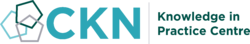## Welcome

Welcome to the CKN Knowledge in Practice Centre (KPC). The KPC is a resource for learning and applying scientific knowledge to the practice of composites manufacturing. As you navigate around the KPC, refer back to the information on this right-hand pane as a resource for understanding the intricacies of composites processing and why the KPC is laid out in the way that it is. The following video explains the KPC approach:

## Understanding Composites Processing

The Knowledge in Practice Centre (KPC) is centered around a structured method of thinking about composite material manufacturing. From the top down, the heirarchy consists of:

The way that the material, shape, tooling & consumables and equipment (abbreviated as MSTE) interact with each other during a process step is critical to the outcome of the manufacturing step, and ultimately critical to the quality of the finished part. The interactions between MSTE during a process step can be numerous and complex, but the Knowledge in Practice Centre aims to make you aware of these interactions, understand how one parameter affects another, and understand how to analyze the problem using a systems based approach. Using this approach, the factory can then be developed with a complete understanding and control of all interactions.

## Interrelationship of Function, Shape, Material & Process

Design for manufacturing is critical to ensuring the producibility of a part. Trouble arises when it is considered too late or not at all in the design process. Conversely, process design (controlling the interactions between shape, material, tooling & consumables and equipment to achieve a desired outcome) must always consider the shape and material of the part. Ashby has developed and popularized the approach linking design (function) to the choice of material and shape, which influence the process selected and vice versa, as shown below:

Within the Knowledge in Practice Centre the same methodology is applied but the process is more fully defined by also explicitly calling out the equipment and tooling & consumables. Note that in common usage, a process which consists of many steps can be arbitrarily defined by just one step, e.g. "spray-up". Though convenient, this can be misleading.

## Workflows

The KPC's Practice and Case Study volumes consist of three types of workflows:

• Development - Analyzing the interactions between MSTE in the process steps to make decisions on processing parameters and understanding how the process steps and factory cells fit within the factory.
• Troubleshooting - Guiding you to possible causes of processing issues affecting either cost, rate or quality and directing you to the most appropriate development workflow to improve the process
• Optimization - An expansion on the development workflows where a larger number of options are considered to achieve the best mixture of cost, rate & quality for your application.